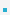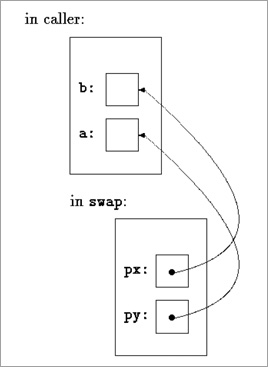2. Pointers and Function ArgumentsSince C passes arguments to functions by value, there is no direct way for the called function to alter    a variable in the calling function. For instance, a sorting routine might exchange two out-of-order    arguments with a function called swap.Call by Value
 int a = 5, b = 7; swap(a, b); void swap(int x, int y) /* WRONG */ {      int temp;      temp = x;      x = y;      y = temp; }
– Because of call by value, swap can't affect the arguments a and b in the routine that called it. The    function above swaps copies of a and b.Call by Reference
 int a = 5, b = 7; swap(&a, &b); /* interchange *px and *py */ void swap(int *px, int *py) {      int temp;      temp = *px;      *px = *py;      *py = temp; }Variables passing out of scopePointer invalid after variable passes out of scope.What is wrong with this code?

 #include char *get_message ( ) {     char msg[] = “Aren't pointers fun?”;     return msg; } int main ( void ) {     char *string = get_message();     puts(string );     return 0; }
– warning: returning address of local variable or temporary

 이전페이지 / 3 / 다음페이지